# Properties of Matter Amole What is Matter Anything

• Slides: 9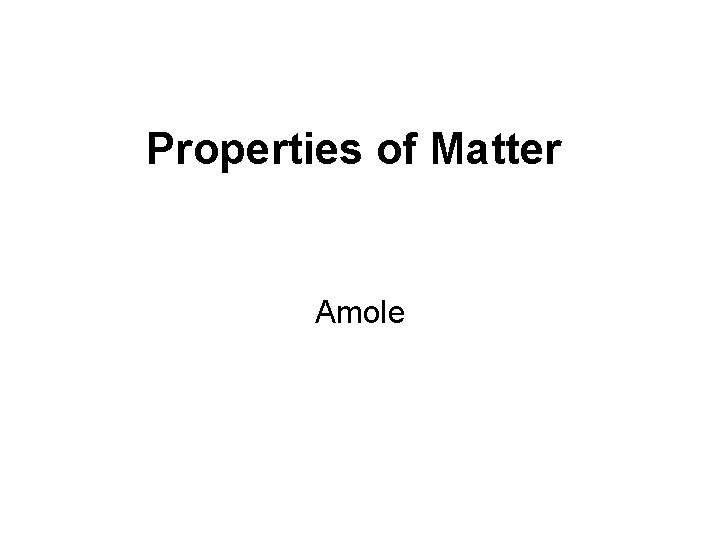Properties of Matter AmoleWhat is Matter? • Anything that has mass and takes up space • Basically, if it has mass and volume…. it’s matter! • Mass and volume are two properties of matter. • So, is air matter? How do you know?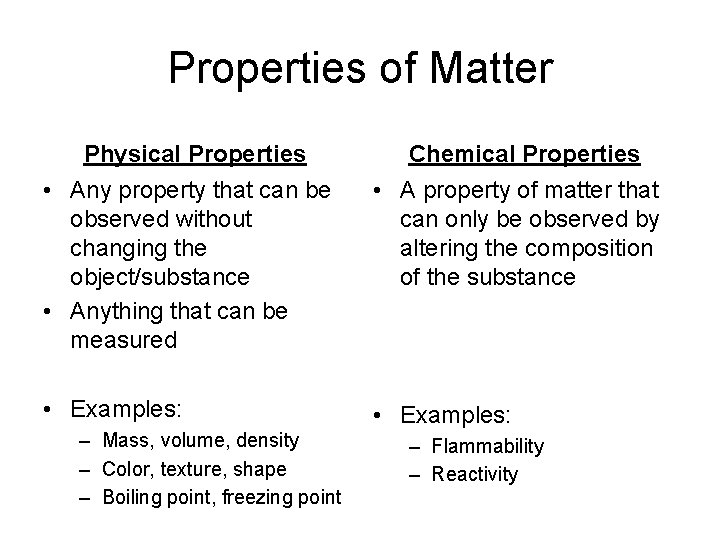Properties of Matter Physical Properties Chemical Properties • Any property that can be observed without changing the object/substance • Anything that can be measured • A property of matter that can only be observed by altering the composition of the substance • Examples: – Mass, volume, density – Color, texture, shape – Boiling point, freezing point – Flammability – Reactivity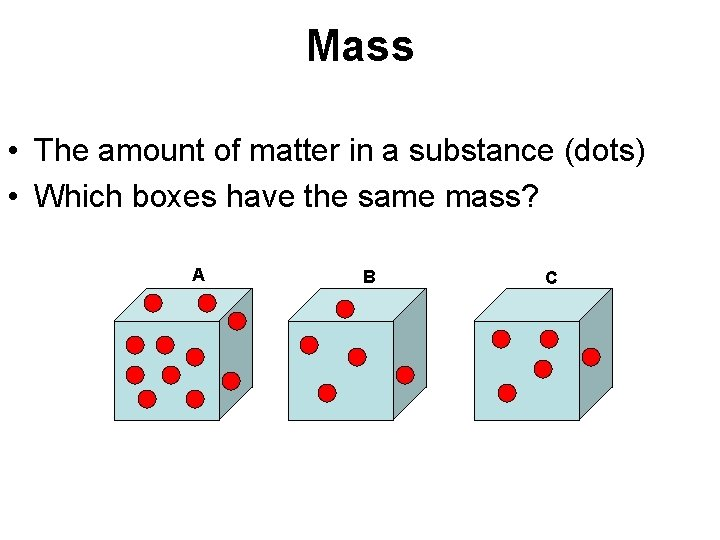Mass • The amount of matter in a substance (dots) • Which boxes have the same mass? A B C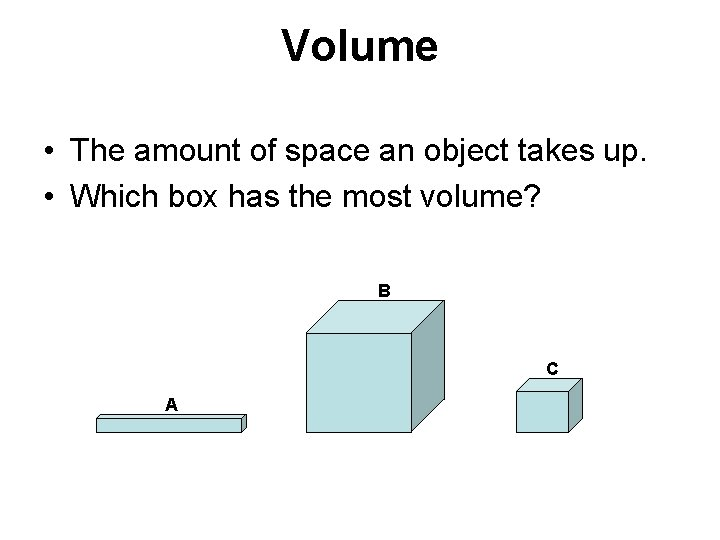Volume • The amount of space an object takes up. • Which box has the most volume? B C ADensity • The amount of mass per unit volume (M/V) • It is a comparison between mass and volume • It describes how “tightly” particles are packed together in a given space. • Which box is more dense? A B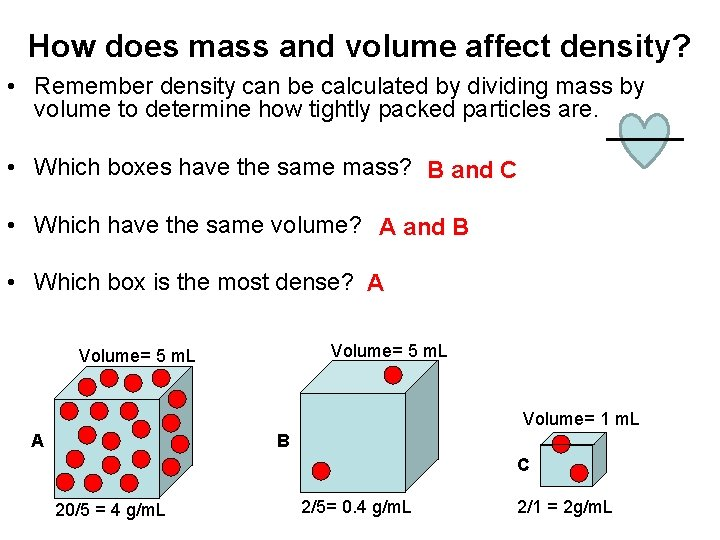How does mass and volume affect density? • Remember density can be calculated by dividing mass by volume to determine how tightly packed particles are. • Which boxes have the same mass? B and C • Which have the same volume? A and B • Which box is the most dense? A Volume= 5 m. L Volume= 1 m. L A B C 20/5 = 4 g/m. L 2/5= 0. 4 g/m. L 2/1 = 2 g/m. LDensity Rule • A substance with a lower density will “float” on top of a substance with a higher density. • Which substance has the highest density? Least dense: Yellow/ Lt. Gray Then: Blue/ Dk. Gray Most dense: Red/ BlackWhat does density have to do with weather? • Air Pressure has a large impact on weather. • Density is affected by pressure. • More pressure forces particles to be packed tighter and increases density. • Also, density dictates the movement of winds. • But…. . how can we be sure air has a density? ? ?